# MAP 3rd Grade Math : Measurement and Data

## Example Questions

### Example Question #1 : Measurement And Data

What is the area of the figure below?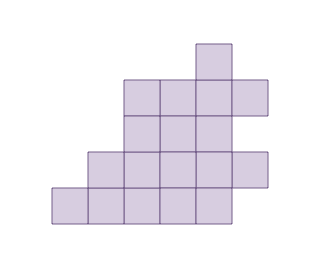Explanation:

Area is the amount of space inside the boarder of a two dimensional shape. This shape is made up of unit squares. We can count the number of squares within the shape to find the area.

There aresquares within the shape, so the area is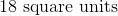### Example Question #1 : Measurement And Data

Megan is working on a science experiment. She needstimes the amount of water that she has shown on the scale below. How much water does she need?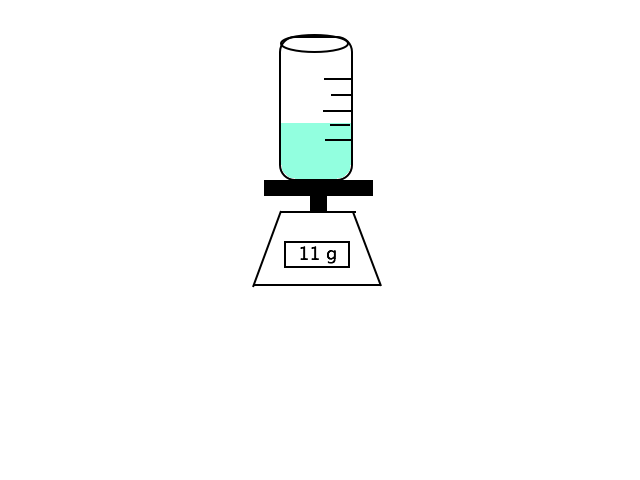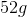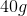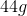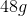Explanation:

Megan has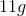of water. If she needstimes that amount, we can multiply to find our answer.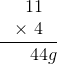### Example Question #11 : Map 3rd Grade Math

What is the perimeter of the rectangle below?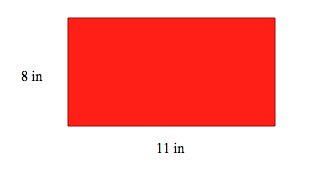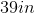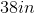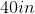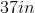Explanation:

The formula for perimeter of a rectangle is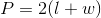To solve for the perimeter we can plug our known values into the equation.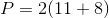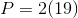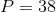### All MAP 3rd Grade Math Resources